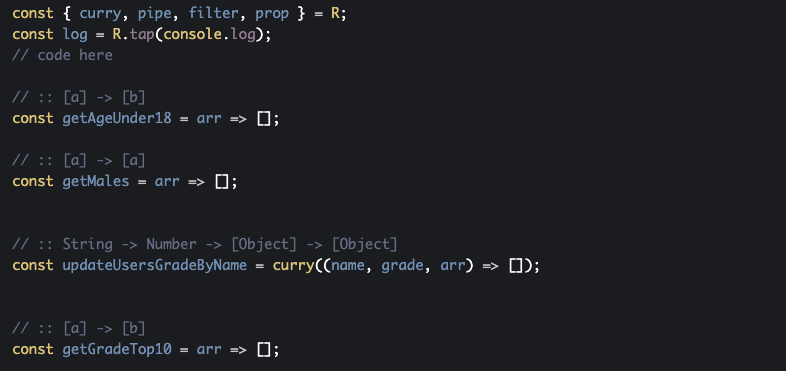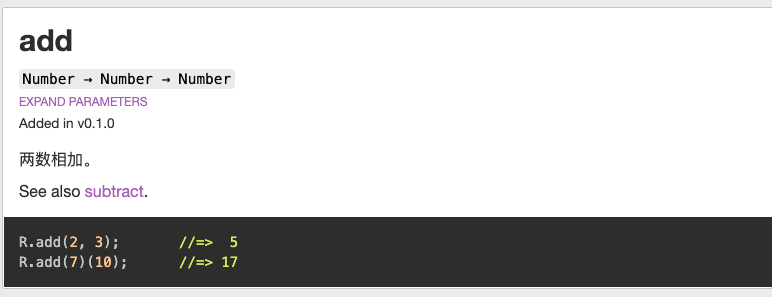# 简明 JavaScript 函数式编程——实践篇

0收藏

``````const split = curry((x, str) => str.split(x));
const join = curry((x, arr) => arr.join(x));
const replaceSpaceWithComma = compose(join(','), split(' '));
const replaceCommaWithDash = compose(join('-'), split(','));``````

``````const split = curry((str, x) => str.split(x));
const replaceSpaceWithComma = compose(join(','), split(R.__, ' '));``````

``````const trace = curry((tip, x) => { console.log(tip, x); return x; });
const lastUppder = compose(toUpperCase, head, trace('after reverse'), reverse);``````

``````const data = [
{
name: 'Peter',
sex: 'M',
age: 18,
},
……
]``````

1. 获取所有年龄小于 18 岁的对象，并返回他们的名称和年龄。
2. 查找所有男性用户。
3. 更新一个指定名称用户的成绩（不影响原数组）。
4. 取出成绩最高的 10 名，并返回他们的名称和分数。

``````// 对象操作（最后一个参数是对象），均会返回新的对象拷贝
R.prop('name')    // 获取对象 name 字段的值
R.propEq('name', '123')   // 判断对象 name 字段是否等于‘123’
R.assoc('name', '123')   // 更新对象的'name'的值为'123'
R.pick(['a', 'd']); //=> {a: 1, d: 4}  // 获取对象某些属性，如果对应属性不存在则不返回
R.pickAll(['a', 'd']); //=> {a: 1, d: 4}  // 获取对象某些属性，如果对应属性不存在则返回`key : undefined`

// 数组操作
R.map(func)  // 传统的 map 操作
R.filter(func)  // 传统的 filter 操作
R.reject(func)  // filter 的补集
R.take(n)    // 取出数组前 n 个元素

// 比较操作
R.equals(a, b)  // 判断 b 是否等于 a
R.gt(2, 1) => true  // 判断第一个参数是否大于第二个参数
R.lt(2, 1) => false // 判断第一个参数是否小于第二个参数

// 排序操作
R.sort(func)    // 根据某个排序函数排序
R.ascend(func)    // 根据 func 转换后的值，生成一个升序比较函数
R.descend(func)    // 根据 func 转换后的值，生成一个降序比较函数
// 例子：
R.sort(R.ascend(R.prop('age')))  // 根据 age 进行升序排序

// 必备函数
R.pipe()   //compose 的反向，从前往后组合
R.compose()  // 从后到前组合
R.curry()  // 柯里化``````Hindly Milner 类型签名

``:: String -> Object````const replace = reg => sub => str => str.replace(reg, sub);``

``````//  replace :: Regex -> String -> String -> String
const replace = reg => sub => str => str.replace(reg, sub);``````

``//  replace :: Regex -> （String -> （String -> String))``

``````// join :: String -> [String] -> String
const join = curry((sep, arr) => arr.join(sep));``````

``````// strLen :: String -> Number
const strLen = str => str.length();``````

``````const joinDash = join('-');
const lengthWithDash = compose(strLen, joinDash);
lengthWithDash(['abc', 'def']);  // 7``````

``````//  map :: (a -> b) -> [a] -> [b]
var map = curry(function(f, xs){
return xs.map(f);
});

//  head :: [a] -> a
var head = function(xs){ return xs; }``````

Pointfree 编程风格

``````// Pointfree  没有出现需要操作的参数
const upperLastItem = compose(toUpperCase, head, reverse);

// 非 Pointfree 出现了需要操作的参数
const upperLastItem = arr => {
const reverseArr = arr.reverse();
}``````

• 无需考虑参数命名：能减轻不少思维负担，毕竟参数命名也是个很费事的过程。
• 关注点集中：你无需考虑数据，只需要把所有的注意力集中在转换关系上。
• 代码精简：可以省去通过中间变量不断的去传递数据的过程。
• 可读性强：一眼就可以看出来数据的整个的转换关系。

• 代码简洁，开发快速：函数式编程大量使用函数的组合，函数的复用率很高，减少了代码的重复，因此程序比较短，开发速度较快。Paul Graham 在《黑客与画家》一书中写道：同样功能的程序，极端情况下，Lisp 代码的长度可能是 C 代码的二十分之一。
• 接近自然语言，易于理解：函数式编程大量使用声明式代码，基本都是接近自然语言的，加上它没有乱七八糟的循环，判断的嵌套，因此特别易于理解。
• 更少的出错概率：因为每个函数都很小，而且相同输入永远可以得到相同的输出，因此测试很简单，同时函数式编程强调使用纯函数，没有副作用，因此也很少出现奇怪的 Bug。

• 性能：函数式编程相对于指令式编程，性能绝对是一个短板，因为它往往会对一个方法进行过度包装，从而产生上下文切换的性能开销。同时，在 JS 这种非函数式语言中，函数式的方式必然会比直接写语句指令慢（引擎会针对很多指令做特别优化）。就拿原生方法 map 来说，它就要比纯循环语句实现迭代慢 8 倍。
• 资源占用：在 JS 中为了实现对象状态的不可变，往往会创建新的对象，因此，它对垃圾回收（Garbage Collection）所产生的压力远远超过其他编程方式。这在某些场合会产生十分严重的问题。
• 递归陷阱：在函数式编程中，为了实现迭代，通常会采用递归操作，为了减少递归的性能开销，我们往往会把递归写成尾递归形式，以便让解析器进行优化。但是众所周知，JS 是不支持尾递归优化的（虽然 ES6 中将尾递归优化作为了一个规范，但是真正实现的少之又少，传送门）
• ……
因此，在性能要求很严格的场合，函数式编程其实并不是太合适的选择。

……帖子
视频
声望
粉丝
最近发布
社区精华内容